# PSAT Math : Arcs and Intercept

## Example Questions

← Previous 1 3 4

### Example Question #1 : Circles

A circle has a center at (5,5) and a radius of 2. If the format of the equation for the circle is (x+A)2+(y+B)2=C, what is C?

3

5

2

1

4

4

Explanation:

The circle has a center at (5,5) and a radius of 2. Therefore, the equation is (x+5)2+(y+5)2=22, or (x+5)2+(y+5)2=4.

### Example Question #2 : Circles

If the center of a circle is at (0,4) and the diameter of the circle is 6, what is the equation of that circle?

x2 + (y-4)2 = 36

x2 + y2 = 9

(x-4)2 + y2 = 36

(x-4)2 + y2 = 9

x2 + (y-4)2 = 9

x2 + (y-4)2 = 9

Explanation:

The formula for the equation of a circle is:

(x-h) 2 + (y-k)2 = r2

Where (h,k) is the center of the circle.

h = 0 and k = 4

and diameter = 6 therefore radius = 3

(x-0) 2 + (y-4)2 = 32

x2 + (y-4)2 = 9

### Example Question #3 : Circles

Circle A is given by the equation (x – 4)2 + (y + 3)2 = 29. Circle A is shifted up five units and left by six units. Then, its radius is doubled. What is the new equation for circle A?

(x – 2)2 + (y + 2)2 = 58

(x + 2)2 + (y – 2)2 = 116

(x + 2)2 + (y – 2)2 = 58

(x – 10)2 + (y + 8)2 = 58

(x – 10)2 + (y + 8)2 = 116

(x + 2)2 + (y – 2)2 = 116

Explanation:

The general equation of a circle is (x – h)2 + (y – k)2 = r2, where (h, k) represents the location of the circle's center, and r represents the length of its radius.

Circle A first has the equation of (x – 4)2 + (y + 3)2 = 29. This means that its center must be located at (4, –3), and its radius is √29.

We are then told that circle A is shifted up five units and then left by six units. This means that the y-coordinate of the center would increase by five, and the x-coordinate of the center would decrease by 6. Thus, the new center would be located at (4 – 6, –3 + 5), or (–2, 2).

We are then told that the radius of circle A is doubled, which means its new radius is 2√29.

Now, that we have circle A's new center and radius, we can write its general equation using (x – h)2 + (y – k)2 = r2.

(x – (–2))2 + (y – 2)2 = (2√29)2 = 22(√29)2 = 4(29) = 116.

(x + 2)2 + (y – 2)2 = 116.

The answer is (x + 2)2 + (y – 2)2 = 116.

### Example Question #2 : How To Find The Equation Of A Circle

Which of the following equations describes all the points (x, y) in a coordinate plane that are five units away from the point (–3, 6)?

(x – 3)2 + (y + 6)2 = 25

y + 6 = 5 – (x – 3)2

(x + 3)2 + (y – 6)2 = 25

(x – 3)2 – (y + 6)2 = 25

(x – 3)2 + (y + 6)2 = 5

(x + 3)2 + (y – 6)2 = 25

Explanation:

We are trying to find an equation for all of the points that are the same distance (5 units) from (–3, 6). The locus of all points equidistant from a single point is a circle. In other words, we need to find an equation of a circle. The center of the circle will be (–3, 6), and the radius, which is the distance from (–3,6), will be 5.

The standard form of a circle is given below:

(x – h)2 + (y – k)2 = r2, where the center is located at (h, k) and r is the length of the radius.

In this case, h will be –3, k will be 6, and r will be 5.

(x – (–3))2 + (y – 6)2 = 52

(x + 3)2 + (y – 6)2 = 25

The answer is (x + 3)2 + (y – 6)2 = 25.

### Example Question #1 : How To Find The Equation Of A Circle

What is the equation for a circle of radius 12, centered at the intersection of the two lines:

y1 = 4x + 3

and

y2 = 5x + 44?

(x - 41)2 + (y - 161)2 = 144

(x - 22)2 + (y - 3)2 = 12

(x + 41)2 + (y + 161)2 = 144

(x - 3)2 + (y - 44)2 = 144

(x + 41)2 + (y + 161)2 = 144

Explanation:

To begin, let us determine the point of intersection of these two lines by setting the equations equal to each other:

4x + 3 = 5x + 44; 3 = x + 44; –41 = x

To find the y-coordinate, substitute into one of the equations.  Let's use y1:

y = 4 * –41 + 3 = –164 + 3 = –161

The center of our circle is therefore: (–41, –161).

Now, recall that the general form for a circle with center at (x0, y0) is:

(x - x0)2 + (y - y0)2 = r2

For our data, this means that our equation is:

(x + 41)2 + (y + 161)2 = 122 or (x + 41)2 + (y + 161)2 = 144

### Example Question #1 : Circles

The diameter of a circle has endpoints at points (2, 10) and (–8, –14). Which of the following points does NOT lie on the circle?

(–8, 10)

(–8,–12)

(9,3)

(–15, –7)

(2, –14)

(–8,–12)

Explanation: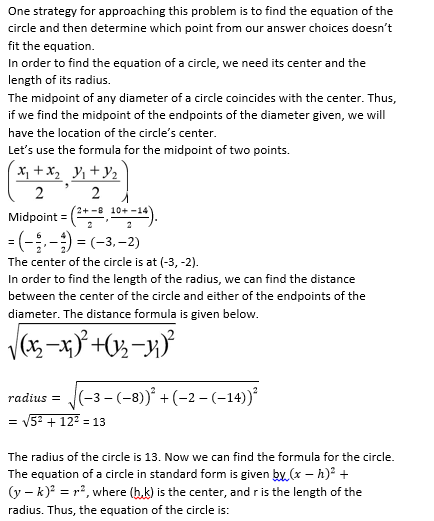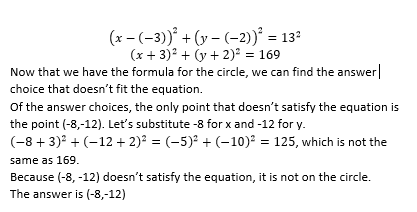### Example Question #1 : How To Find The Equation Of A Circle

A circle exists entirely in the first quadrant such that it intersects the-axis at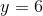.  If the circle intersects the-axis in at least one point, what is the area of the circle?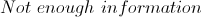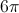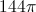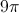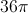Explanation:

We are given two very important pieces of information.  The first is that the circle exists entirely in the first quadrant, the second is that it intersects both the- and-axis.

The fact that it is entirely in the first quadrant means that it cannot go past the two axes.  For a circle to intersect the-axis in more than one point, it would necessarily move into another quadrant. Therefore, we can conclude it intersects in exactly one point.

The intersection of the circle with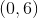must also be tangential, since it can only intersect in one point.  We can thus conclude that the circle must have both- and- intercepts equal to 6 and have a center of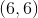.

This leaves us with a radius of 6 and an area of: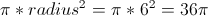### Example Question #1 : Circles

We have a square with length 2 sitting in the first quadrant with one corner touching the origin. If the square is inscribed inside a circle, find the equation of the circle.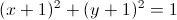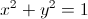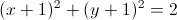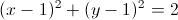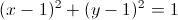Explanation:

If the square is inscribed inside the circle, in means the center of the circle is at (1,1). We need to also find the radius of the circle, which happens to be the length from the corner of the square to it's center.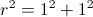Now use the equation of the circle with the center and.

We get### Example Question #1 : How To Find The Equation Of A Circle

What is the radius of a circle with the equation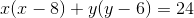?Explanation:

We need to expand this equation to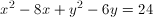and then complete the square.

This brings us to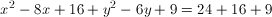.

We simplify this to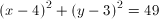.

### Example Question #1 : How To Find The Equation Of A Circle

A circle has its origin at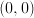. The point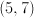is on the edge of the circle. What is the radius of the circle?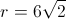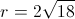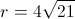There is not enough information to answer this question.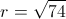Explanation:

The radius of the circle is equal to the hypotenuse of a right triangle with sides of lengths 5 and 7.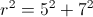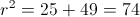This radical cannot be reduced further.

← Previous 1 3 4

### All PSAT Math Resources